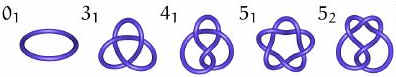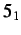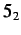Next: Properties of knots Up: text_knot Previous: How do we work

# Classifying different knotsOne of the first objectives mathematicians wished to achieve in working with knots was formulating tables of distinct knots. To be able to classify knots, it is easiest if we work with only one projection (or representation) of each knot to avoid duplication.

First, we must "simplify" the knot as much as possible. This means we use the Reidemeister moves (from the previous section) to get as few crossings in the knot as possible. Once we simplify the knot so that we cannot remove any further crossings, the knot is classified by the number of crossings that remain. For example, the trefoil knot is classified by its fewest number of crossings - three (see the diagram above).

Sometimes it is possible to have more than one knot with the same number of crossings. In this case, we usually use subscripts to denote different knots with the same number of crossings, such as theand. knots in the diagram above.Next: Properties of knots Up: text_knot Previous: How do we work
Supriya Garg 2004-05-02